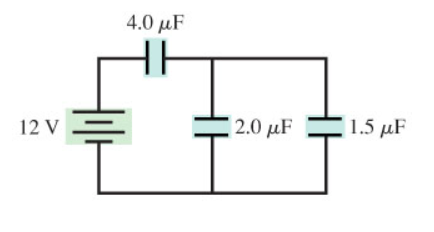# Problem: Part A. What is the equivalent capacitance for the circuit of the figure? (Figure 1) Express your answer to two significant figures and include the appropriate units. Part B. How much charge flows through the battery as the capacitors are being charged? Express your answer to two significant figures and include the appropriate units.

###### FREE Expert Solution

Equitavent capacitance for capacitors in series:

$\overline{){{\mathbf{C}}}_{\mathbf{e}\mathbf{q}}{\mathbf{=}}\frac{{\mathbf{C}}_{\mathbf{1}}{\mathbf{C}}_{\mathbf{2}}}{{\mathbf{C}}_{\mathbf{1}}\mathbf{+}{\mathbf{C}}_{\mathbf{2}}}}$

Equitavent capacitance for capacitors in paralle:

$\overline{){{\mathbf{C}}}_{\mathbf{e}\mathbf{q}}{\mathbf{=}}{{\mathbf{C}}}_{{\mathbf{1}}}{\mathbf{+}}{{\mathbf{C}}}_{{\mathbf{2}}}{\mathbf{+}}{\mathbf{.}}{\mathbf{.}}{\mathbf{.}}}$

Charge is given by:

$\overline{){\mathbf{Q}}{\mathbf{=}}{\mathbf{C}}{\mathbf{V}}}$

Part A

2.0 μF and 1.5 μ capacitors are in parallel.

92% (95 ratings)###### Problem DetailsPart A. What is the equivalent capacitance for the circuit of the figure? (Figure 1) Express your answer to two significant figures and include the appropriate units.

Part B. How much charge flows through the battery as the capacitors are being charged? Express your answer to two significant figures and include the appropriate units.